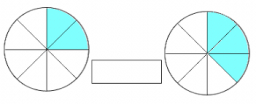Add/subtract the following similar fraction. Express the answer in the lowest terms if possible. Write your answer inside the box:

5/12 + 6/12

x =  11/12

### Step-by-step explanation:Did you find an error or inaccuracy? Feel free to write us. Thank you!

Showing 1 comment:
Benice
I want to know the answer to each subject

Tips for related online calculators
Need help calculating sum, simplifying, or multiplying fractions? Try our fraction calculator.